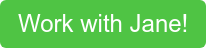The symbol “dx” comes up everywhere in calculus. For example:But what is “dx” really? It’s more than just notation! In this post, we’ll explore the meaning of “dx” and try to get a better understanding of some of the symbols that we often see in calculus.

## Calculus as the study of infinitesimal change

Calculus is the study of continuous change, or infinitesimal change. To get an idea of what this means, let’s consider the following: suppose that you’re running a race. You begin running at time x=0 seconds and then track your displacement as a function f(x). Your displacement function f(x) is then a continuous function that varies over time.

Suppose now that you wanted to know your speed at time x=10 seconds. That is a calculus question since you are looking for a rate of change at one particular time. How could you do this? Well, you might estimate your speed at time t=10 as our average speed between time x=10 and x=11, which can be expressed asReally, what you want to do is to take the limit as the size of your time interval goes to zero. That is, you want to take an infinitesimally small change in x. Then, by the definition of a derivative, your speed at x=10 seconds isWe should think of h going to zero as taking smaller and smaller increases in x when we take the average speed from time x=10 to time x=10+h.

This is where “dx” comes in.We can see this if we revisit our speed example from earlier. When computing our derivativethe bottom of this fraction is (10+h)-10 as h goes to zero, which is an infinitesimally small change in x. We might therefore think of the denominator as h goes to zero as dx. If we let y=f(x), then the numerator of this fraction is f(10+h)-f(10) as h goes to zero, which is an infinitesimally small change in y, or dy. Putting this all together, we recover the notationThat is, the derivative of f(x) is the quotient of an infinitesimal change in y over an infinitesimal change in x. Put more precisely, it is exactly the limit of the change in y over the change in x over smaller and smaller changes in x. The “dx” and “dy” notation just captures this limiting procedure and expresses it as an infinitesimal change in x or y instead.

## “dx” as seen in integralsAnother way to think about this is that in our integral, we are summing up infinitesimally thin rectangles with height f(x) and width dx to compute our signed area precisely.

Hopefully the symbol “dx” is a little less mysterious now!

Jane obtained her BA in mathematics from Princeton University, where she graduated summa cum laude and received a departmental award for her thesis. She then earned her PhD in mathematics from MIT, where she was partially supported by a graduate research fellowship from the NSF. For her work in leading undergraduate math recitations at MIT, she was awarded the Housman award for excellence in teaching. Moving forward, she is continuing to focus on both teaching and research as a postdoctoral fellow at Indiana University Bloomington.

Throughout her academic career, Jane has enjoyed teaching and mentoring students, and has sought out a variety of teaching experiences. As an undergraduate, she was a course assistant in undergraduate math courses and taught engineering enrichment lessons in local elementary schools. During her graduate years, she taught recitations for both calculus and linear algebra at MIT. She also mentored middle and high school girls at Girls’ Angle, a local after school math club, where she worked with small groups of students on everything from homework and test prep, to enrichment activities, to math research projects.  She has also spent her summers teaching at various math and science summer programs for high school and college students.Check out some of our previous blog posts related to mathematics below!

Tags: math